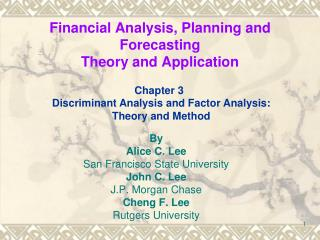# Financial Analysis, Planning and Forecasting Theory and Application - PowerPoint PPT PresentationDownload PresentationFinancial Analysis, Planning and Forecasting Theory and Application

Financial Analysis, Planning and Forecasting Theory and ApplicationDownload Presentation## Financial Analysis, Planning and Forecasting Theory and Application

- - - - - - - - - - - - - - - - - - - - - - - - - - - E N D - - - - - - - - - - - - - - - - - - - - - - - - - - -
##### Presentation Transcript

1. Financial Analysis, Planning and ForecastingTheory and Application Chapter 3 Discriminant Analysis and Factor Analysis: Theory and Method By Alice C. Lee San Francisco State University John C. Lee J.P. Morgan Chase Cheng F. Lee Rutgers University

2. Outline • 3.1 Introduction • 3.2 Important concepts of linear algebra Linear combination and its distribution Vectors, matrices, and their operations Linear-equation system and its solution • 3.3 Two-group discriminant analysis • 3.4 k-group discriminant analysis • 3.5 Factor analysis and principal-component analysis Factor score Factor loadings • 3.6 Summary • Appendix 3A. Discriminant analysis and dummy regression analysis • Appendix 3B. Principal-component analysis

3. 3.2 Important concepts of linear algebra • Linear combination and its distribution • Vectors, matrices, and their operations • Linear-equation system and its solution

4. 3.2 Important concepts of linear algebra (3.1) (3.1′) (3.2a) (3.2b)

5. 3.2 Important concepts of linear algebra (3.2b′) (3.2b′′)

6. 3.2 Important concepts of linear algebra

7. 3.2 Important concepts of linear algebra Step 1: Multiply A’ by B Step 2: Multiply C by A Linear Equation System and its Solution

8. 3.2 Important concepts of linear algebra

9. 3.2 Important concepts of linear algebra (3.5) (3.6)

10. 3.2 Important concepts of linear algebra (3.7) (3.8)

11. 3.2 Important concepts of linear algebra

12. 3.2 Important concepts of linear algebra

13. 3.2 Important concepts of linear algebra

14. 3.2 Important concepts of linear algebra Note to instructor: The numbers with red circle are different from those in the text.

15. Extra Example to show how simultaneous equation system can be solve by matrix inversion method The simultaneous equation (a) can be written as matrix form as equation (b)Then we can solve this equation system by matrix inversion.

16. We know Please note that this is one of three methods can be used to solve simultaneous equation system. Other two methods are substitution method and Cramer rule method. These two methods have been taught in high school algebra. In practice, matrix method is the best method to solve large equation systems, such as portfolio analysis (see Chapter 7).

17. Cramer’s Rule

18. 3.3 Two-group Discriminant Analysis where B = DD′, between-group variance; C = Within-group variance; A = Coefficient vector representing the coefficients of Eq. (3.8); E = Ratio of the weighted between-group variance to the pooled within variance. (3.12)

19. 3.3 Two-group discriminant analysis TABLE 3.1 Roster of liquidity and leverage ratios For two groups with two predictors and a “dummy” criterion variable Y.

20. 3.3 Two-group discriminant analysis (3.13) (3.14) Var(x1i)a1 + Cov(x1i , x2i) a2 = Cov(x1i , yi) (3.15a) Cov(x1i , x2i) a1 + Var(x2i)a2 = Cov(x2i , yi) (3.15b)

21. 3.3 Two-group discriminant analysis

22. 3.3 Two-group discriminant analysis

23. 3.3 Two-group discriminant analysis

24. 3.3 Two-group discriminant analysis

25. 3.3 Two-group discriminant analysis

26. 3.3 Two-group discriminant analysis

27. 3.3 Two-group discriminant analysis

28. 3.4 k-group discriminant analysis (3.17) (3.18)

29. 3.4 k-group discriminant analysis (3.20a) (3.20b) (3.20c) (3.20r)

30. 3.4 k-group discriminant analysis (3.21a) (3.21b) Where = Prior probability of being classified as bankrupt, = Prior probability of being classified as non-bankrupt, = Conditional probability of being classified as non- bankrupt when, in fact, the firm is bankrupt, = Conditional probability of being classified as bankrupt when, in fact, the firm is non-bankrupt, = Cost of classifying a bankrupt firm as non-bankrupt, = Cost of classifying a non-bankrupt firm as bankrupt.

31. 3.5 Factor analysis and principal-component analysis • Factor score • Factor loadings

32. 3.5 Factor analysis and principal-component analysis (3.22) (3.23) (3.24)

33. 3.6 Summary • In this chapter, method and theory of both discriminant analysis and factor analysis needed for determining useful financial ratios, predicting corporate bankruptcy, determining bond rating, and analyzing the relationship between bankruptcy avoidance and merger are discussed in detail. Important concepts of linear algebra-linear combination and matrix operations- required to understand both discriminant and factor analysis are discussed.

34. Appendix 3A. Discriminant analysis and dummy regression analysis (3.A.1) (3.A.2) where

35. Appendix 3A. Discriminant analysis and dummy regression analysis (3.A.3) (3.A.2a)

36. Appendix 3A. Discriminant analysis and dummy regression analysis (3.A.4) (3.A.5)

37. Appendix 3A. Discriminant analysis and dummy regression analysis (3.A.6) (3.A.7) (3.A.8)

38. Appendix 3A. Discriminant analysis and dummy regression analysis BA = ECA. (3.A.9) (1 + E)BA = E(B + C)A or (3.A.10)

39. Appendix 3A. Discriminant analysis and dummy regression analysis (3.A.11) (3.A.12) (3.A.l’) (3.A.13)

40. Appendix 3A. Discriminant analysis and dummy regression analysis (3.A.l4a) (3.A.l4b) (3.A.l5)

41. Appendix 3A. Discriminant analysis and dummy regression analysis (3.A.l6) .

42. Appendix 3B. Principal-component analysis

43. Appendix 3B. Principal-component analysis （3.B.1） （3.B.2） （3.B.3）